# Samacheer Kalvi 6th Maths Solutions Term 1 Chapter 4 Geometry Additional Questions## Tamilnadu Samacheer Kalvi 6th Maths Solutions Term 1 Chapter 4 Geometry Additional Questions

Question 1.
From the given figure, name the parallel lines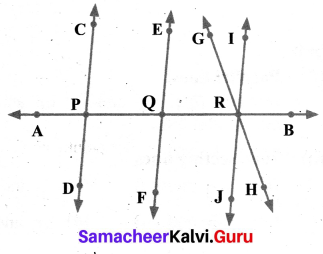Solution:
(i) Parallel Lines:
$$\overrightarrow{\mathrm{CD}}$$ and $$\overrightarrow{\mathrm{EF}}$$ ; $$\overrightarrow{\mathrm{CD}}$$ and $$\overrightarrow{\mathrm{IJ}}$$ ; $$\overrightarrow{\mathrm{EF}}$$ and $$\overrightarrow{\mathrm{IJ}}$$ are parallel lines.
(ii) Intersecting lines:
(a) $$\overrightarrow{\mathrm{AB}} \text { and } \overrightarrow{\mathrm{CD}}$$
(b) $$\overrightarrow{\mathrm{AB}} \text { and } \overrightarrow{\mathrm{EF}}$$
(c) $$\overrightarrow{\mathrm{AB}} \text { and } \overrightarrow{\mathrm{GH}}$$
(d) $$\overrightarrow{\mathrm{AB}} \text { and } \overrightarrow{\mathrm{IJ}}$$
(e) $$\overrightarrow{\mathrm{GH}} \text { and } \overrightarrow{\mathrm{IJ}}$$
(iii) Points of Intersection:
P, Q and R are the points of intersection.

Question 2.
(a) Name the line segments in the figure.
(b) Is Q, the endpoint of each line segment?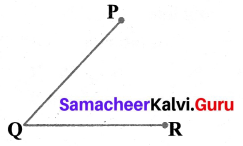Solution:
(a) $$\overline{\mathrm{QP}} \text { and } \overline{\mathrm{QR}}$$ are the line segments
(b) Yes, Q is the end point of each line segment

Question 3.
How many lines can pass through
(a) one given point
(b) two given points.
Solution: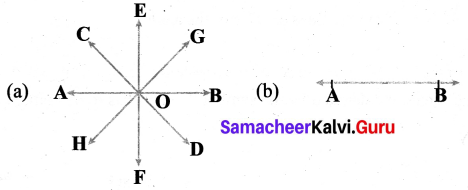(a) An infinite number of lines can pass through one given point.
(b) Exactly one and only one line can pass through two given points.

Question 4.
A line contains how many points?
(a) minimum?
(b) maximum?
Solution:
(a) A line contains a minimum of two points.
(b) A line contain a maximum of infinitely many points.Question 5.
Write the (a) maximum and (b) the minimum number of point of intersection of three lines.
Solution: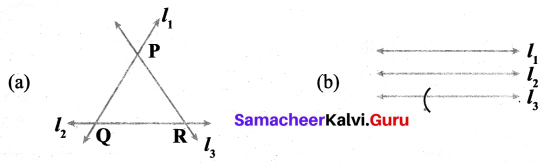Maximum – 3 points of intersection
Minimum – No point of intersection

Fill in the blanks.

Question 6.
Complementary angle of 20° is _____
Solution:
70°

Question 7.
The supplementary angle of 90° is _____
Solution:
90°

Question 8.
78°, 12°, ______
Solution:
Complementary angle

Question 9.
∠ABD =?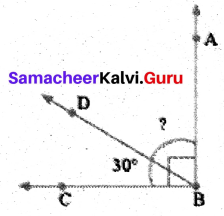Solution:
On Sum of complementary angles = 90°
∠ABC = 90°
∠CBD = 30°
∠ABD = ∠ABC – ∠DBC = 90° – 30° = 60°
∠ABD = 60°
Complementary angle of 30° = 60°

Question 10.
In the following figure, name the angles.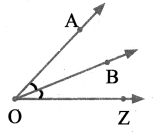Solution:
∠AOB, ∠BOZ, ∠AOZ

Question 11.
Write the alternate name of the angle ∠XYZ in the given figure.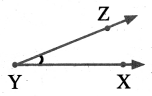Solution:
∠Y or ∠ZYXQuestion 12.
Draw the diagram of two angles having only one common point.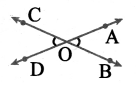Solution:
∠COD and ∠AOB have the point ‘O’ in common

Question 13.
What are the supplementary and complementary angles of 60°?
Solution:
Supplementary angle is 120°
Complementary angle is 30°

Question 14.
How many lines can you draw passing through three collinear points? Draw the figure also.
Solution:
Only one.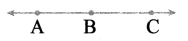Question 15.
Write the maximum number of lines that can pass through a single point.
Solution:
Infinite.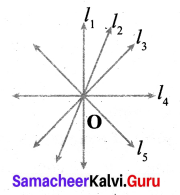Question 16.
Use a protractor to draw an angle 45°.
Solution: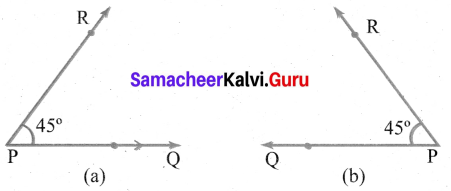Construction:
1. Drawn the base ray PQ.
2. Placed the centre of the protractor at the vertex P. Lined up the ray $$\overrightarrow{\mathrm{PQ}}$$ with the 0° line. Then drawn and labelled a pointed (R) at the 45° mark on the inner scale (a) anticlockwise and (b) outer scale (clockwise)
3. Removed the protractor and drawn at $$\overrightarrow{\mathrm{PR}}$$ to complete the angle
Now ∠P = ∠QPR = ∠RPQ = 45°.